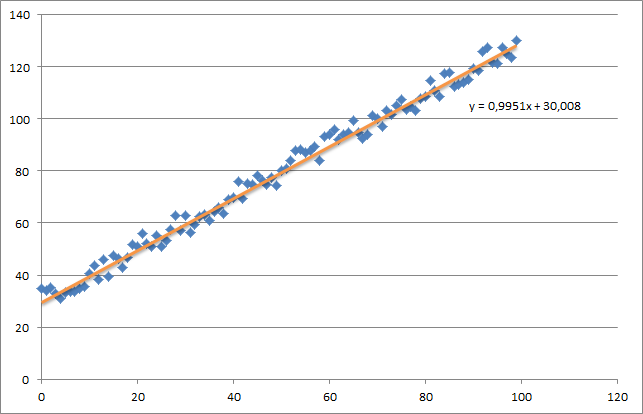2 views

temp=np.array(np.zeros_like(theta,float))

for i in range(0,num_it):

h=np.dot(X_norm,theta)

#temp[j]=theta[j]-(alpha/m)*(  np.sum( (h-y)*X_norm[:,j][np.newaxis,:] )  )

temp=theta-(alpha/m)*(np.sum(h-y))

temp=theta-(alpha/m)*(np.sum((h-y)*X_norm[:,1]))

theta=temp

return theta

X_norm,mean,std=featureScale(X)

#length of X (number of rows)

m=len(X)

X_norm=np.array([np.ones(m),X_norm])

n,m=np.shape(X_norm)

num_it=1500

alpha=0.01

theta=np.zeros(n,float)[:,np.newaxis]

X_norm=X_norm.transpose()

print theta

My theta from the above code is 100.2 100.2, but it should be 100.2 61.09 in Matlab which is correct.

by (33.1k points)

Your question looks quite unclear. I’d like to rephrase it for better understanding:

• To calculate the hypothesis h = X * theta
• To calculate the loss = h - y
• For the squared cost (loss^2)/2m
• Calculate the gradient = X' * loss / m
• Update the parameters theta = theta-alpha * gradient

In your case, you have confused m with n. Here m denotes the number of examples in your training set, not the number of features.

Improved Cod

e:

impo

rt nu

mpy as np

import

random

def gradientDescent(x, y, theta, alpha, m, numIterations):

xTrans = x.transp

ose()

for

i in range(0, numIterations):

hypothesis = np.dot(x, theta)

loss = hypothesis - y

cost = np.sum(loss ** 2) / (2 * m)

print("Iteration %d | Cost: %f" % (i, cost))

e

/ m

# update

theta = theta - alpha * gradient

return theta

def genData(numPoints, bias, varianc

e):

x

= np.zeros(shape=(numPoints,

2))

y

= np.zeros(shape=numPoints)

# basically a straight

line

for i in range(0, numPoints):

# bias feature

x[i] = 1

x[i] = i

# our target variable

y[i] = (i + bias) + random.uniform(0, 1) * var

iance

return x, y

x, y = genData(100

, 25, 10)

m, n

= np.shape(x)

numIterations= 100000

alpha = 0.000

5

theta = np.one

s(n)

theta = gradientDescent(x, y, theta, alpha, m, numIterations)

print(the

ta)

I cr

eate a small random dataset which should look like this:Linear Regression

You need to take care of the intuition of the regression using gradient descent. Once you are done with a complete batch pass over your data X, you need to reduce the m-losses of every iteration to a single weight update. In this case, this is the average of the sum over the gradients, thus the division by m.

If you track the convergence and optimize the gradient, then the result over each iteration looks like:

Iteration 99997 | Cost: 47883.706462

Iteration 99998 | Cost: 47883.706462

Iteration 99999 | Cost: 47883.706462

[ 29.25567368   1.01108458]

We passed the bias into the first column, the first theta value denotes the bias weight.